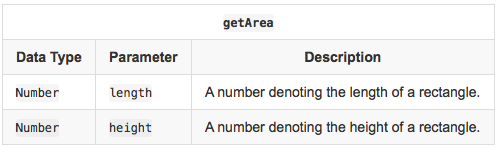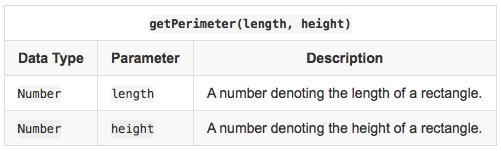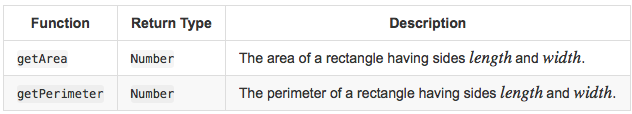## Algorithm

Hacker rank Problem Name: 10 Days of JavaScript - Day 1: Arithmetic Operators

Objective

In this challenge, we practice using arithmetic operators. Check out the attached tutorial for resources.

Complete the following functions in the editor below:

1. `getArea(length, width)`: Calculate and return the area of a rectangle having sides Length and width
2. `getPerimeter(length, width)`: Calculate and return the perimeter of a rectangle having sides Length and width

The values returned by these functions are printed to stdout by locked stub code in the editor.

Input FormatConstraints

• 1 <= Lengt, width <= 1000
• Length and width

are scaled to at most three decimal places.

Output FormatSample Input 0

```3
4.5
```

Sample Output 0

```13.5
15
```

Explanation 0

The area of the rectangle is

Length * width = 3 * 4.5 = 13.5
The perimeter of the rectangle is

2. (Length + width) - 2 (3 * 4.5) = 15

## Code Examples

### #1 Code Example with Javascript Programming

```Code - Javascript Programming```

``````
'use strict';

process.stdin.resume();
process.stdin.setEncoding('utf-8');

let inputString = '';
let currentLine = 0;

process.stdin.on('data', inputStdin => {
inputString += inputStdin;
});

process.stdin.on('end', _ => {
inputString = inputString.trim().split('\n').map(string => {
return string.trim();
});

main();
});

return inputString[currentLine++];
}

function getArea(length, width) {
let area;
area = length * width;
return area;
}

function getPerimeter(length, width) {
let perimeter;
perimeter=2 * (length + width);
return perimeter;
}

``````
Copy The Code &

Input

cmd
3 4.5

Output

cmd
13.5 15